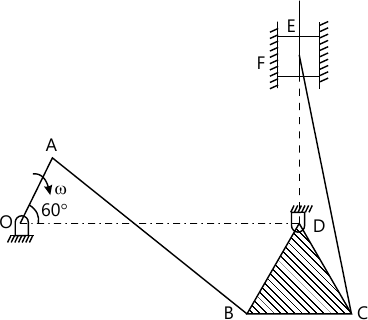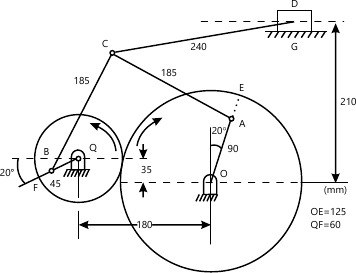MORE IN Theory of Machines - 1
MU Mechanical Engineering (Semester 4)
Theory of Machines - 1
May 2012
Total marks: --
Total time: --
INSTRUCTIONS
(1) Assume appropriate data and state your reasons
(2) Marks are given to the right of every question
(3) Draw neat diagrams wherever necessary

Any four.
1(a) Explain any four(a)Self closure and force closure
5 M
1(b) Law of gearing
5 M
1(c) Chordal action in chains
5 M
1(d) Kennedy's Theorem
5 M
1(e) Peauceller's Mechanism.
5 M

2(a) The link length of a quardric cycle chain are l1=10cm,l2=20cm,l3=30cm and l4=40cm.find all the inversions.
6 M
2(b) The mechanism shows in the figure 1,the various links are OA =100mm,AB=350mm,BC=170mm,BD=CD=140mm,OD=400mm,CE=350mm and Locate all the instantaneous centres,if the slider E moves up wit velocity of 5m/s,determine the speed of rotation of the crank OA.14 M

3(a) An Andrew variable stroke engine mechanism is shown in the figure2, The crank OA rotates r.p.m.find (i)The linear acceleration of slider D.
The angular acceleration of links AC,BC and CD.16 M
3(b) Show all directions of Coriolli's components of acceleration.
4 M

4(a) Derive an expression for maximum efficiency of Helical gear pair in mesh at any instant.
6 M
4(b) A horizontal double acting steam engine has cylinder diameter 220mm,length of stroke 360mm and runs at 240r.p.m. The length of connecting rod is 720mm.The mass of the reciprocating part is 100kg.steam is admitted at a pressure of 6 bar gauge and cut off takes place at $\dfrac{1}{4}\$th of the stroke.After this expansion takes place according to the law pV=constant.The exhaust iis at pressure of -0.80bar gauge. Determine the torque exerted on the shaft when crank has turned through 75° from inner dead centre,Neglect the effect of clearance and assume atmospheric pressure as 1bar.
14 M

5(a) For the two shafts connected by Hooke's joint,derive an expression for $\dfrac{w_{2}}{w_{1}}\$and $\dfrac{dw_{2}}{dt}\$where w_{2} is the angular velocity of driving shaft.
10 M
5(b) An open belt connects two pulleys,the smaller pulley being 400mm diameter.Angle of contact on the smaller pulley is 160° and co-efficient of friction between belt and pulley is 0.25.Determine which of the following alternatives would be more effective in increasing the power transmission capacity
Increase the initial tension by 10%.
Increasing the coefficient of friction by 10% Using suitable dressing system.
Increase the angle of contact by 10% with Use of idler pulley.
10 M

6(a) Explain work energy principle.
5 M
6(b) A bicycle driven single acting air compressor is being used for spray painting application.the data on capability of human being indicate that a man can provide energy in the following order.
1000w for 5seconds.
150w for next 5seconds.
100w for next 30minutes
75w for next 10 minutes
750w for next 5 seconds.
None for the 19minutes and 45seconds.The cycle is than repeated. Assuming a 90% mechanical efficiency.Calculate the average power out put at 100 r.p.m,If a speed variation of 15% is allowed,determine moment of inertia of flywheel.
10 M
6(c) What do you mean by 'Stub tooth system"?What are advantages of system?
5 M

7(a) The following data relate to two meshing involute gears:
Number of teeth on wheel =60
Pressure angle =20°
Gear ratio =1.5
Speed of gear wheel =100r.p.m
Module=8mm
The addendum on each wheel is such that the path of approaches and path of recess on each side are 40% of the maximum possible length each.Determine the addendum for each wheel and length of arc of contact and contact ratio.
10 M
7(b) What are straight line motion mechanism? How are they classified?
5 M
7(c) With a neat sketch explain Ackerma's steering Gear Mechanism.
5 M

More question papers from Theory of Machines - 1